# Red-Black Tree | Set 2 (Insert)

• Difficulty Level : Medium
• Last Updated : 13 Jun, 2022

In the previous post, we discussed the introduction to Red-Black Trees. In this post, insertion is discussed. In AVL tree insertion, we used rotation as a tool to do balancing after insertion. In the Red-Black tree, we use two tools to do the balancing.

1. Recoloring
2. Rotation

Recolouring is the change in colour of the node i.e. if it is red then change it to black and vice versa. It must be noted that the colour of the NULL node is always black. Moreover, we always try recolouring first, if recolouring doesn’t work, then we go for rotation. Following is a detailed algorithm. The algorithms have mainly two cases depending upon the colour of the uncle. If the uncle is red, we do recolour. If the uncle is black, we do rotations and/or recolouring.#### The representation we will be working with is: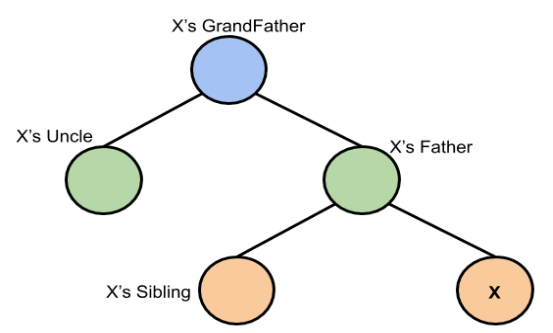This representation is based on X

#### Logic:

First, you have to insert the node similarly to that in a binary tree and assign a red colour to it. Now, if the node is a root node then change its colour to black, but if it is not then check the colour of the parent node. If its colour is black then don’t change the colour but if it is not i.e. it is red then check the colour of the node’s uncle. If the node’s uncle has a red colour then change the colour of the node’s parent and uncle to black and that of grandfather to red colour and repeat the same process for him (i.e. grandfather).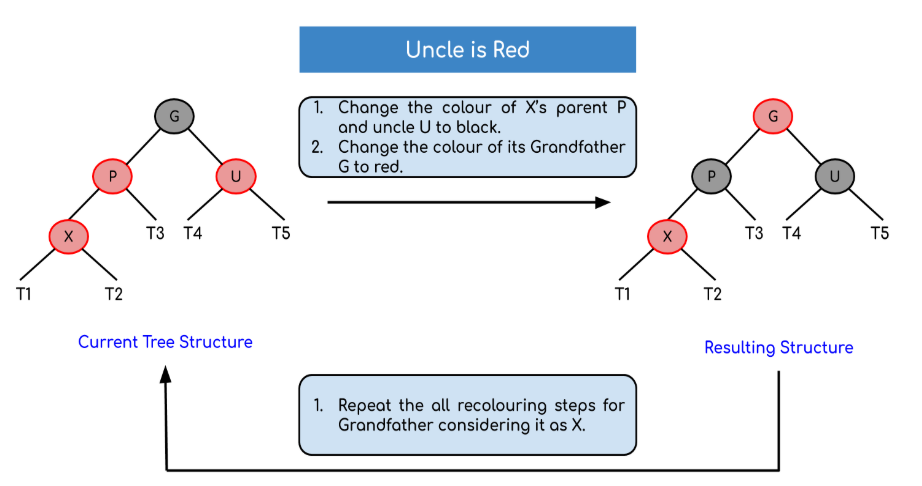But, if the node’s uncle has black colour then there are 4 possible cases:

• Left Left Case (LL rotation):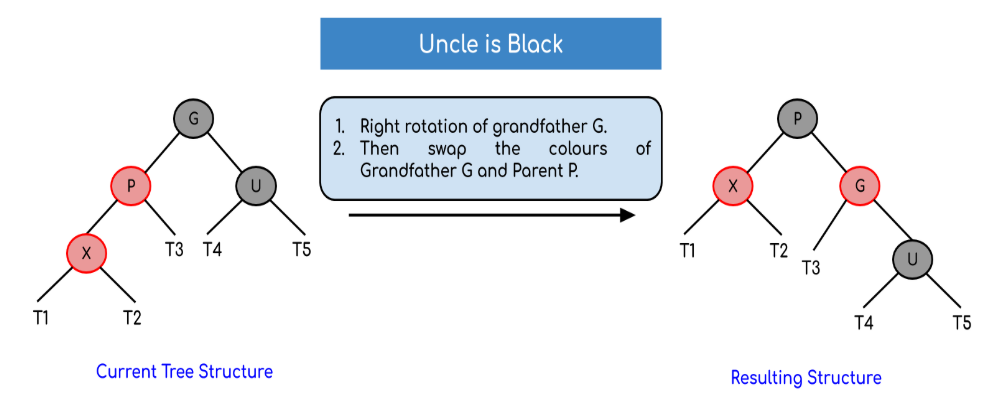• Left Right Case (LR rotation):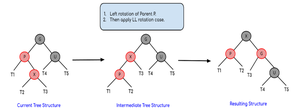• Right Right Case (RR rotation):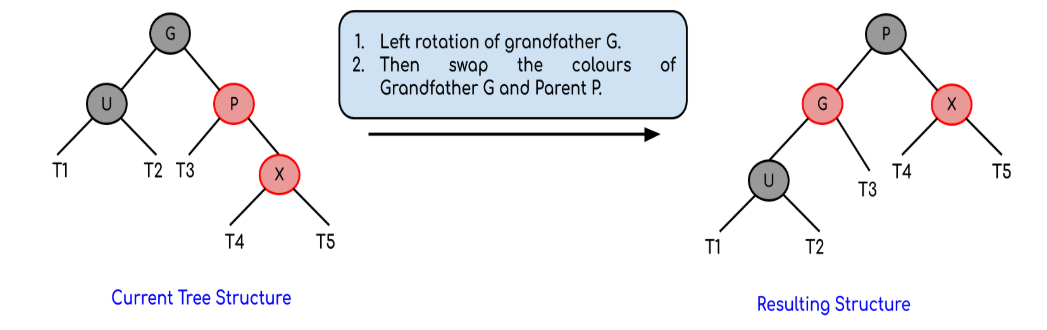• Right Left Case (RL rotation):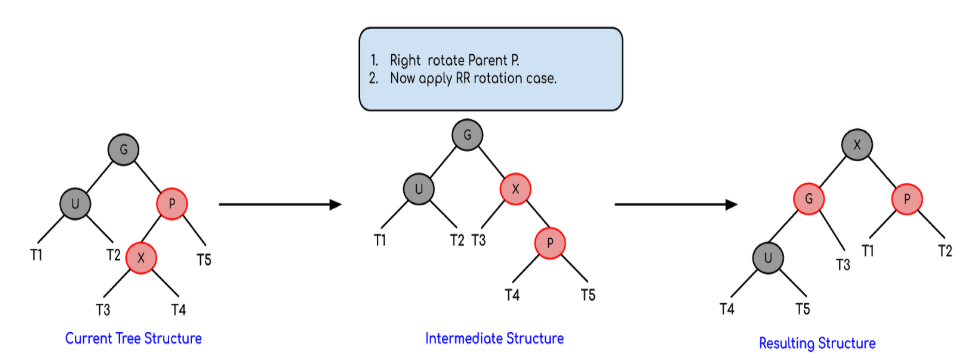Now, after these rotations, if the colours of the nodes are miss matching then recolour them.

#### Algorithm:

Let x be the newly inserted node.

1. Perform standard BST insertion and make the colour of newly inserted nodes as RED.
2. If x is the root, change the colour of x as BLACK (Black height of complete tree increases by 1).
3. Do the following if the color of x’s parent is not BLACK and x is not the root.
a) If x’s uncle is RED (Grandparent must have been black from property 4
(i) Change the colour of parent and uncle as BLACK.
(ii) Colour of a grandparent as RED.
(iii) Change x = x’s grandparent, repeat steps 2 and 3 for new x.
b) If x’s uncle is BLACK, then there can be four configurations for x, x’s parent (p) and x’s grandparent (g) (This is similar to AVL Tree
(i) Left Left Case (p is left child of g and x is left child of p)
(ii) Left Right Case (p is left child of g and x is the right child of p)
(iii) Right Right Case (Mirror of case i)
(iv) Right Left Case (Mirror of case ii)

Example: Creating a red-black tree with elements 3, 21, 32 and 15 in an empty tree.

Solution:When the first element is inserted it is inserted as a root node and as root node has black colour so it acquires the colour black.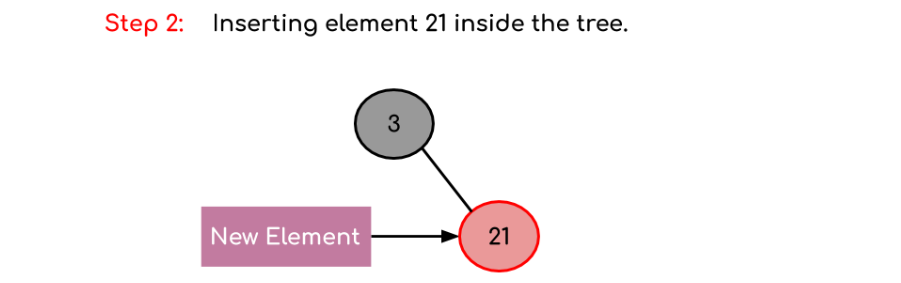The new element is always inserted with a red colour and as 21 > 3 so it becomes the part of the right subtree of the root node.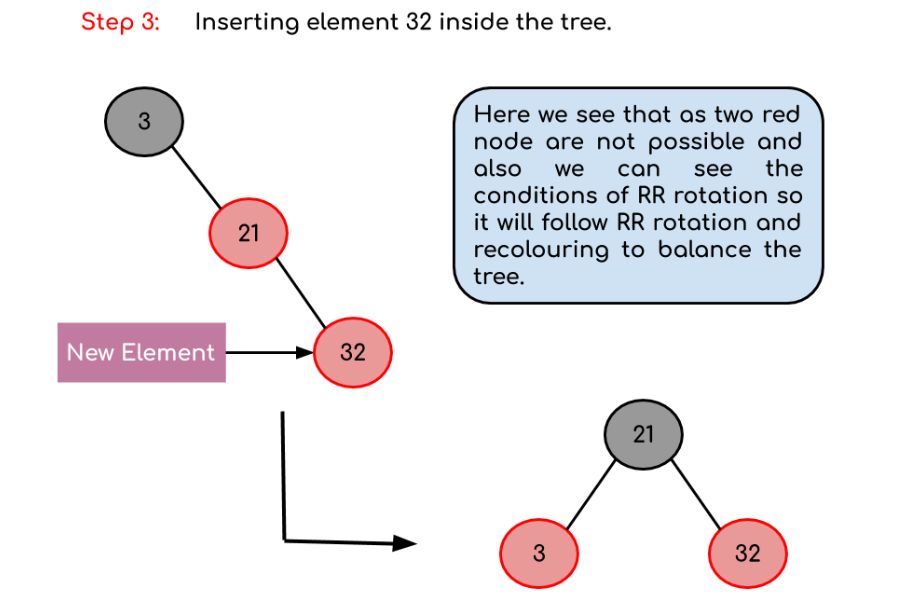Now, as we insert 32 we see there is a red father-child pair which violates the Red-Black tree rule so we have to rotate it. Moreover, we see the conditions of RR rotation (considering the null node of the root node as black) so after rotation as the root node can’t be Red so we have to perform recolouring in the tree resulting in the tree shown above.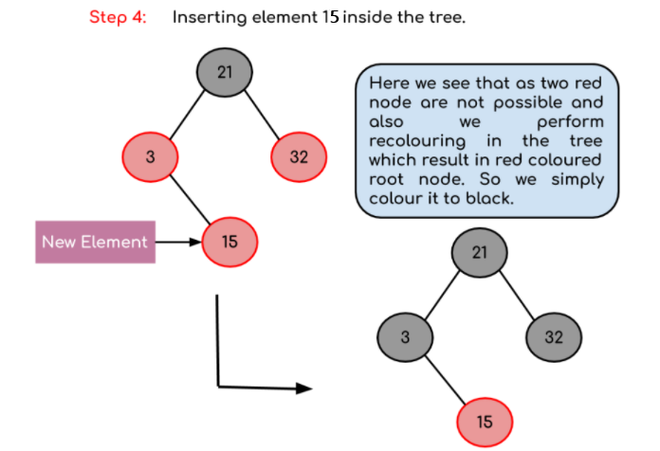Final Tree Structure:The final tree will look like this

Please refer C Program for Red Black Tree Insertion for complete implementation of the above algorithm.

Red-Black Tree | Set 3 (Delete)

## Code for Insertion in Java.

Here is the code written in java for implementation of RED-BLACK Trees

The following code also implements tree insertion as well as tree traversal.

at the end you can visualize the constructed tree too!!!.

## Java

 `/*package whatever //do not write package name here */`   `import` `java.io.*;`   `// considering that you know what are red-black trees here is the implementation in java for insertion and traversal.` `// RedBlackTree class. This class contains subclass for node` `// as well as all the functionalities of RedBlackTree such as - rotations, insertion and ` `// inoredr traversal` `public` `class` `RedBlackTree` `{` `    ``public` `Node root;``//root node` `    ``public` `RedBlackTree() ` `    ``{` `        ``super``();` `        ``root = ``null``;` `    ``}` `    ``// node creating subclass` `    ``class` `Node` `    ``{` `        ``int` `data;` `        ``Node left;` `        ``Node right;` `        ``char` `colour;` `        ``Node parent;`   `        ``Node(``int` `data)` `        ``{` `            ``super``();` `            ``this``.data = data;   ``// only including data. not key` `            ``this``.left = ``null``; ``// left subtree` `            ``this``.right = ``null``; ``// right subtree` `            ``this``.colour = ``'R'``; ``// colour . either 'R' or 'B'` `            ``this``.parent = ``null``; ``// required at time of rechecking.` `        ``}` `    ``}` `    ``// this function performs left rotation` `    ``Node rotateLeft(Node node)` `    ``{` `        ``Node x = node.right;` `        ``Node y = x.left;` `        ``x.left = node;` `        ``node.right = y;` `        ``node.parent = x; ``// parent resetting is also important.` `        ``if``(y!=``null``)` `            ``y.parent = node;` `        ``return``(x);` `    ``}` `    ``//this function performs right rotation` `    ``Node rotateRight(Node node)` `    ``{` `        ``Node x = node.left;` `        ``Node y = x.right;` `        ``x.right = node;` `        ``node.left = y;` `        ``node.parent = x;` `        ``if``(y!=``null``)` `            ``y.parent = node;` `        ``return``(x);` `    ``}`     `    ``// these are some flags.` `    ``// Respective rotations are performed during traceback.` `    ``// rotations are done if flags are true.` `    ``boolean` `ll = ``false``;` `    ``boolean` `rr = ``false``;` `    ``boolean` `lr = ``false``;` `    ``boolean` `rl = ``false``;` `    ``// helper function for insertion. Actually this function performs all tasks in single pass only.` `    ``Node insertHelp(Node root, ``int` `data)` `    ``{` `        ``// f is true when RED RED conflict is there.` `        ``boolean` `f=``false``;` `        `  `        ``//recursive calls to insert at proper position according to BST properties.` `        ``if``(root==``null``)` `            ``return``(``new` `Node(data));` `        ``else` `if``(data

Output

```1
1 4
1 4 6
1 3 4 6
1 3 4 5 6
1 3 4 5 6 7
1 3 4 5 6 7 8
1 2 3 4 5 6 7 8
1 2 3 4 5 6 7 8 9
9

8

7

6

5

4

3

2

1```

My Personal Notes arrow_drop_up
Recommended Articles
Page :